# 使用Xcode + Python进行IOS运动轨迹模拟

## 硬件环境：

MacBook Pro (15-inch, 2017)

iPhone 8 Plus

## 软件环境：

macOS Mojave 10.14.5

iOS 12.4

Xcode 10.2.1

Python 3.7.0

sublime text 3.1.1

# 具体步骤

## 1.使用Xcode创建一个IOS项目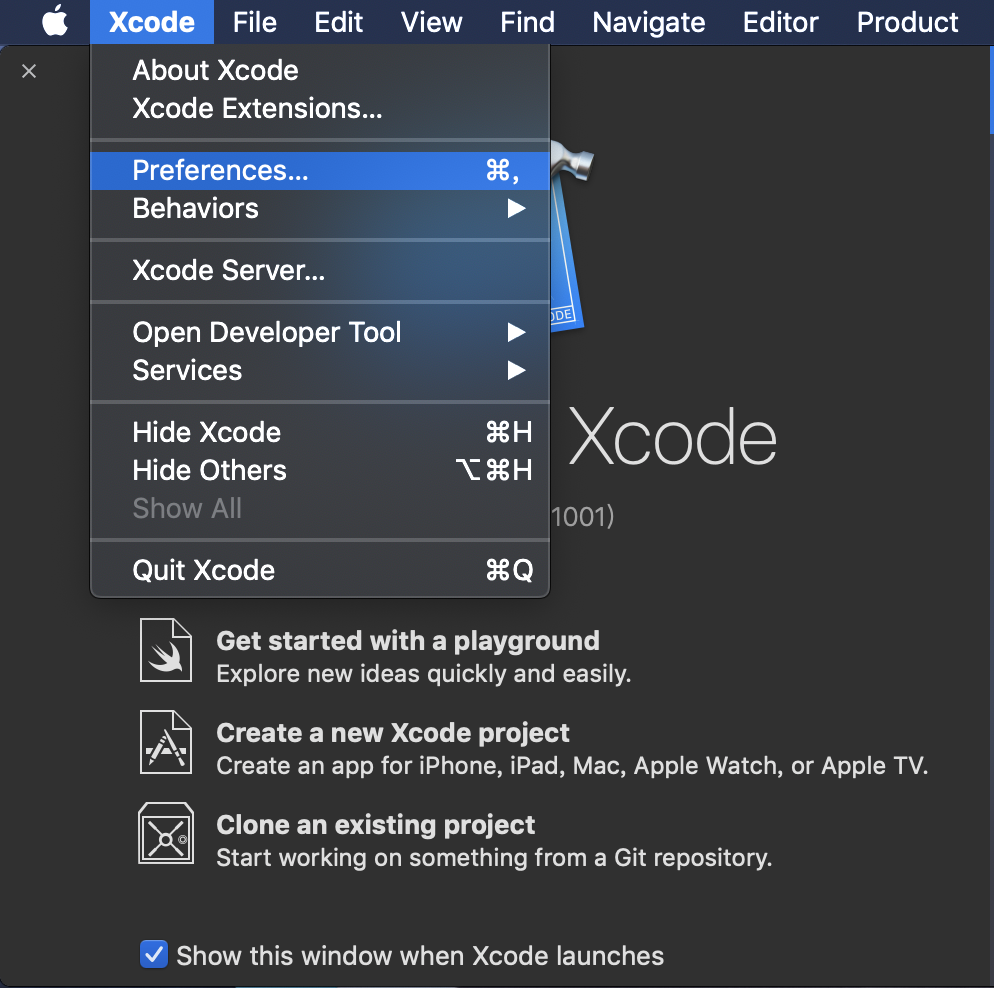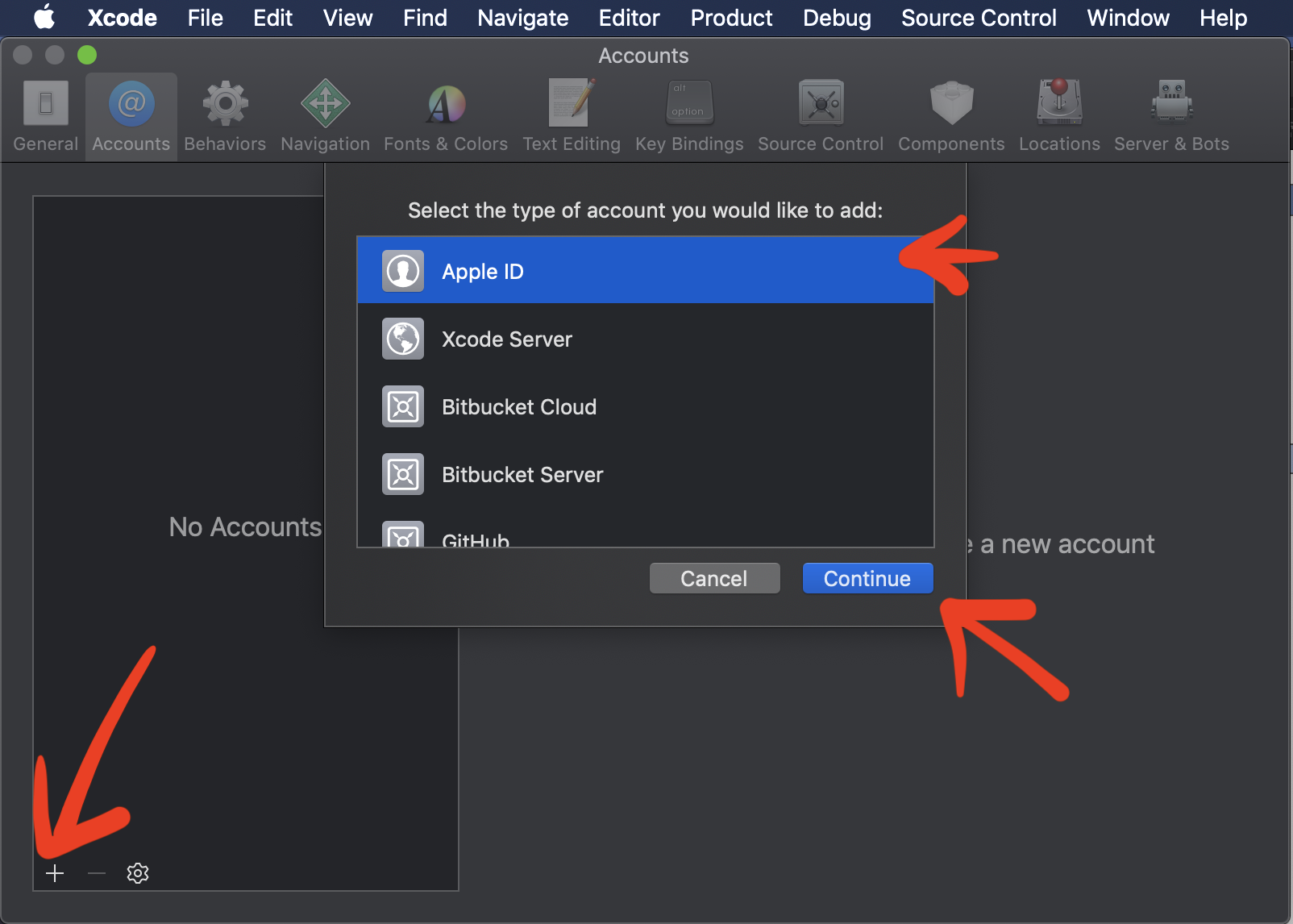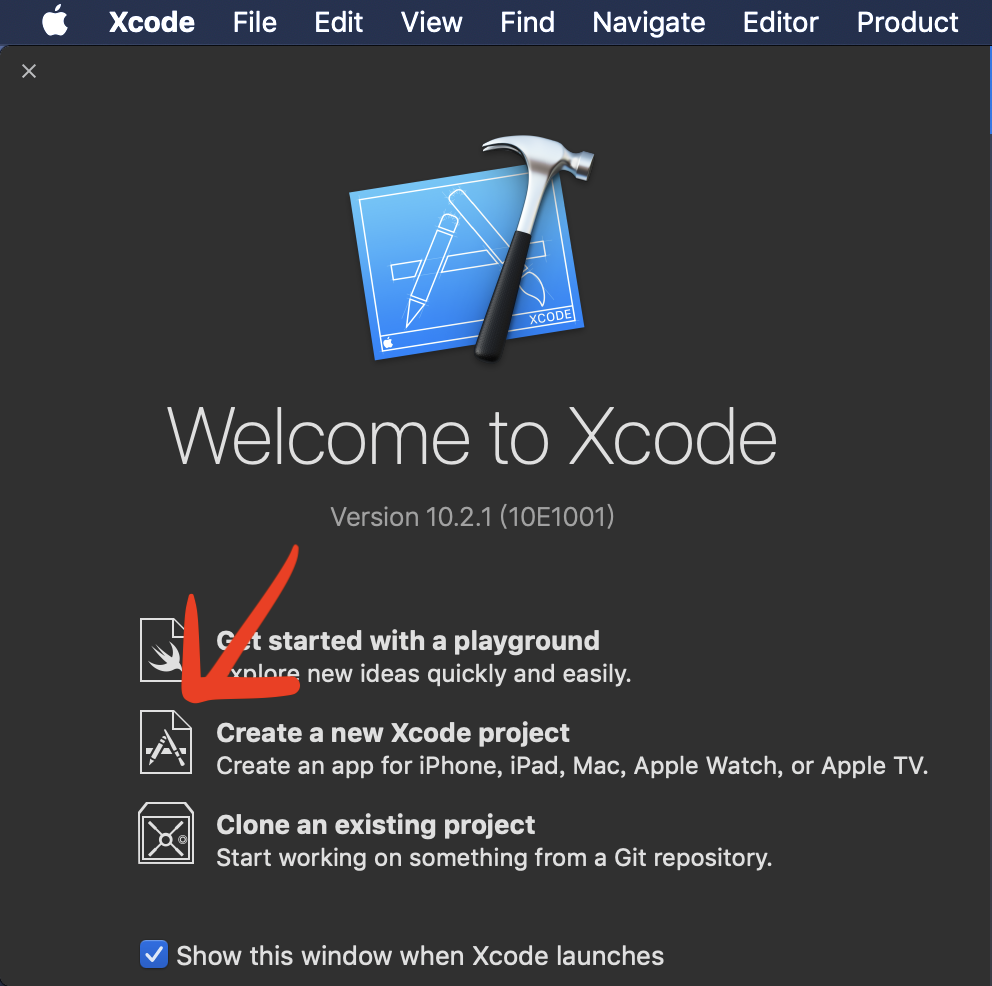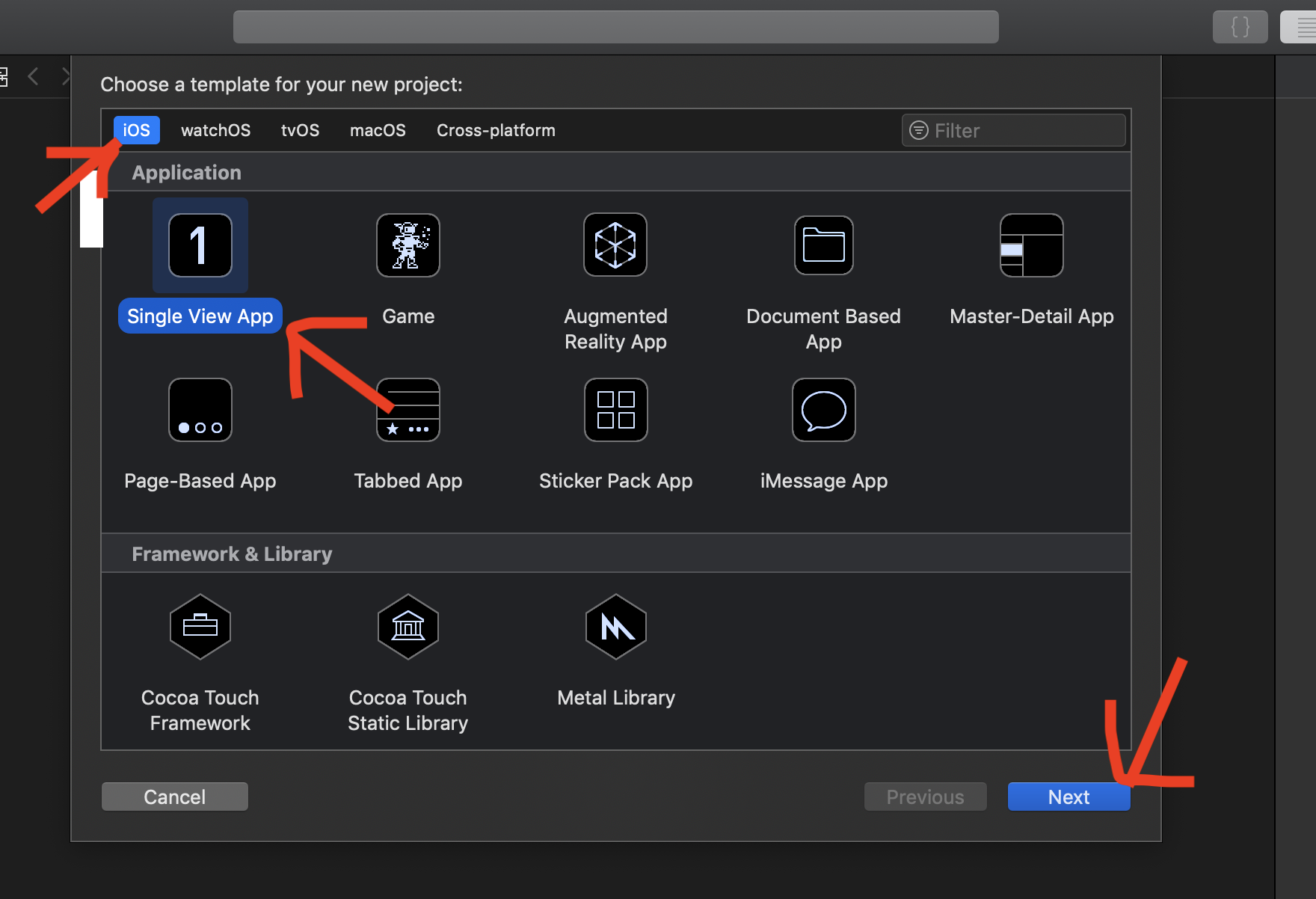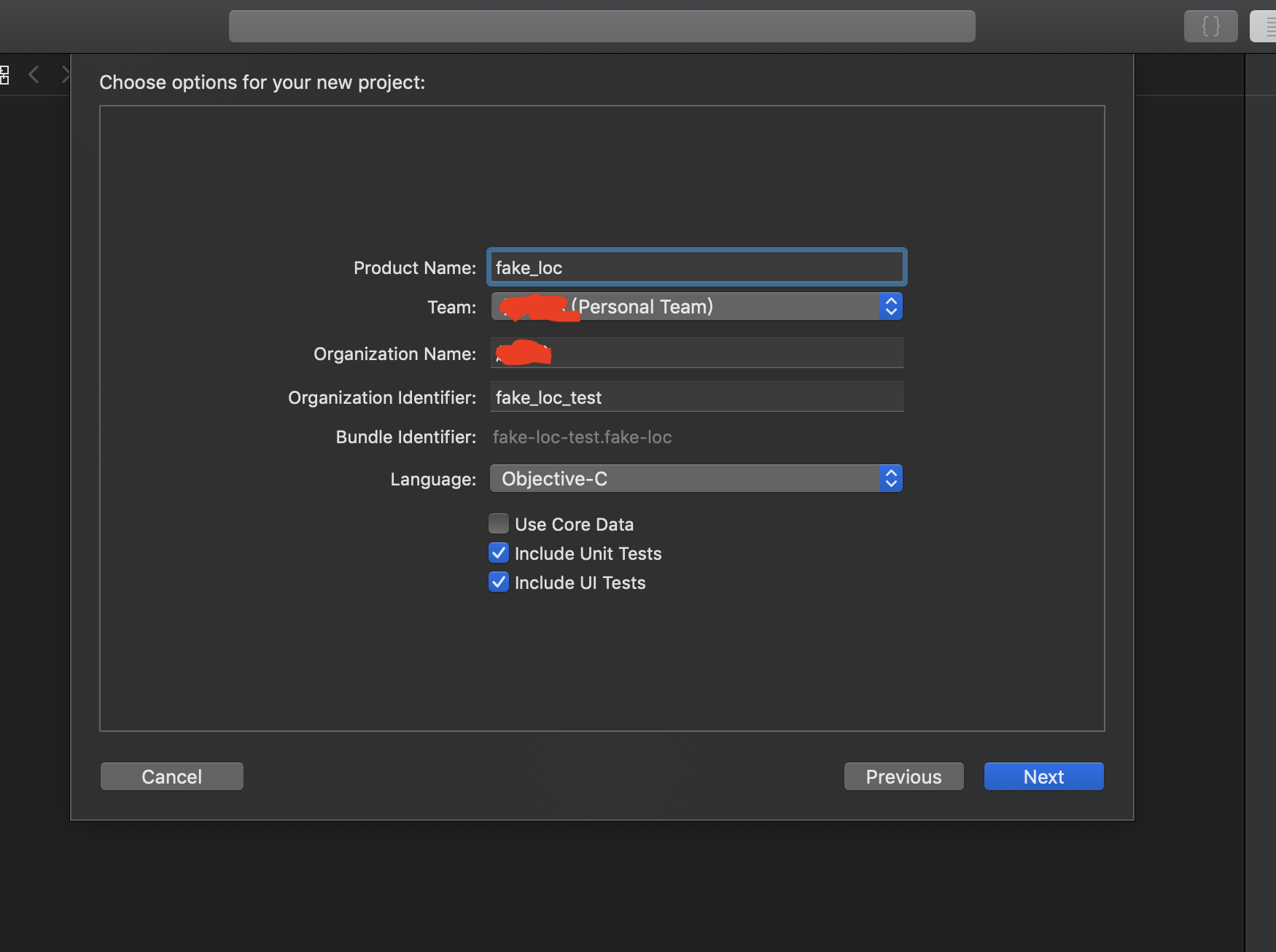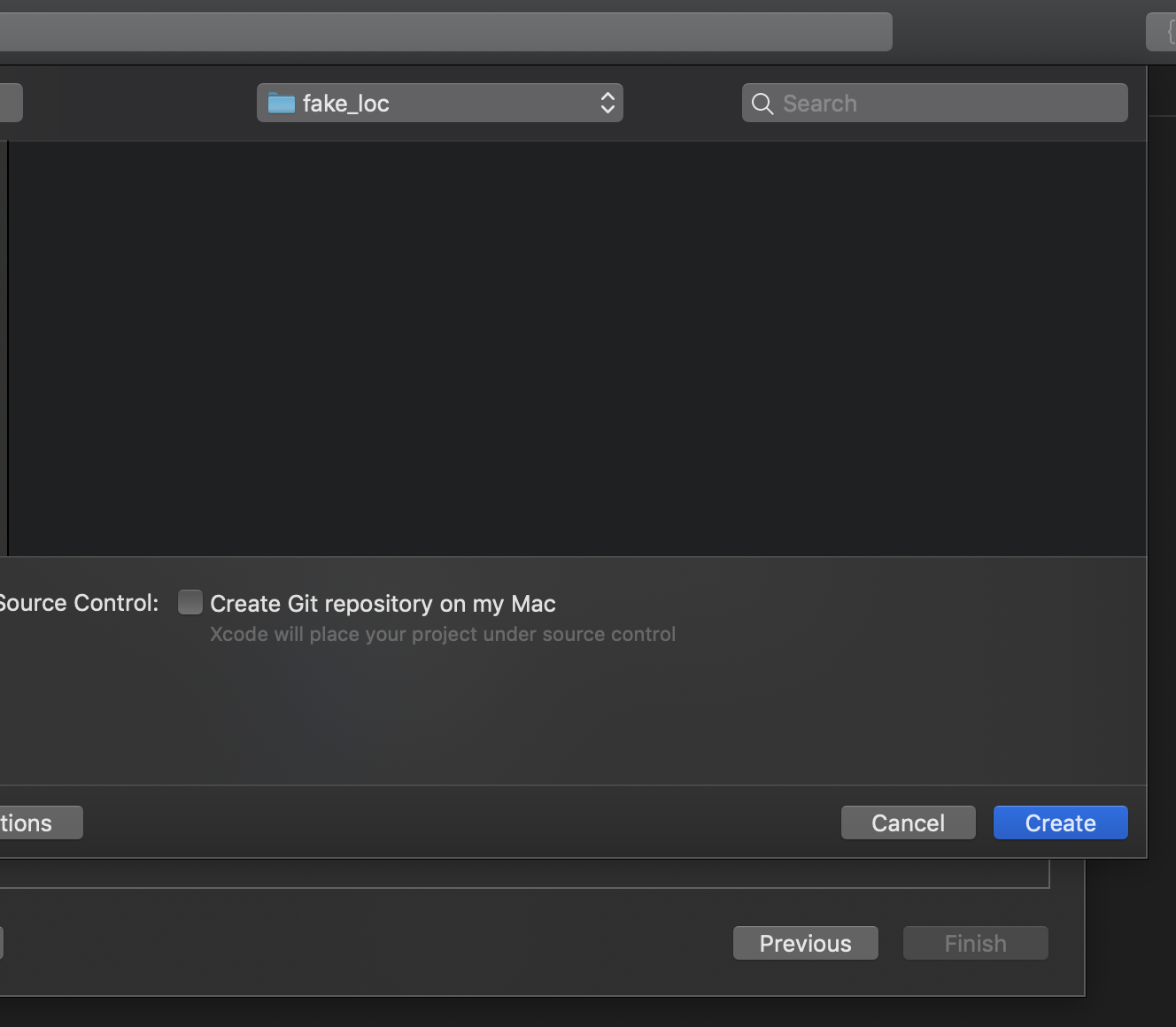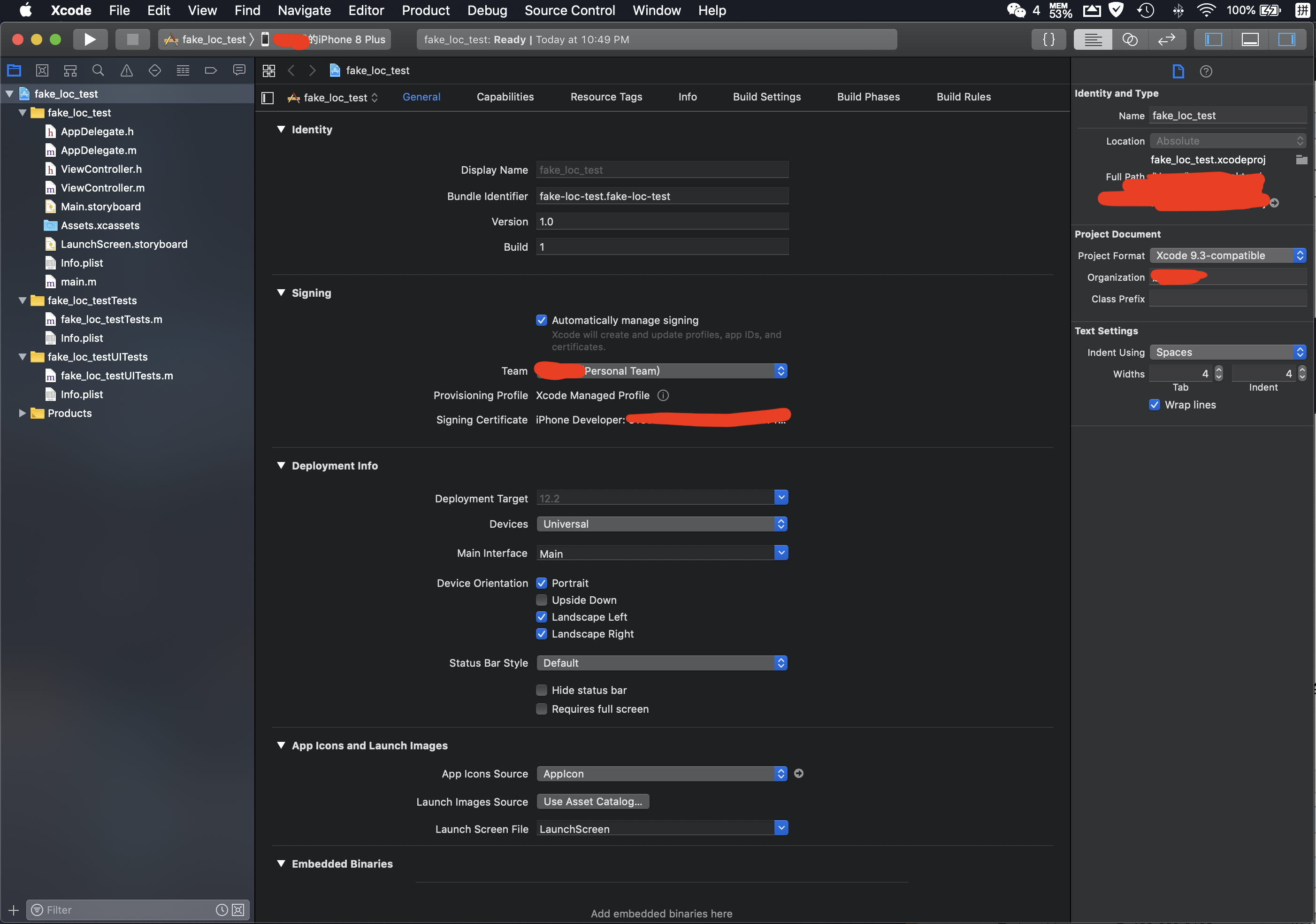## 2.使用Xcode创建一个含有gpx的文件

GPX（GPS eXchange Format，GPS交换格式）是一个XML格式，为应用软件设计的通用GPS数据格式，专门用来存储地理信息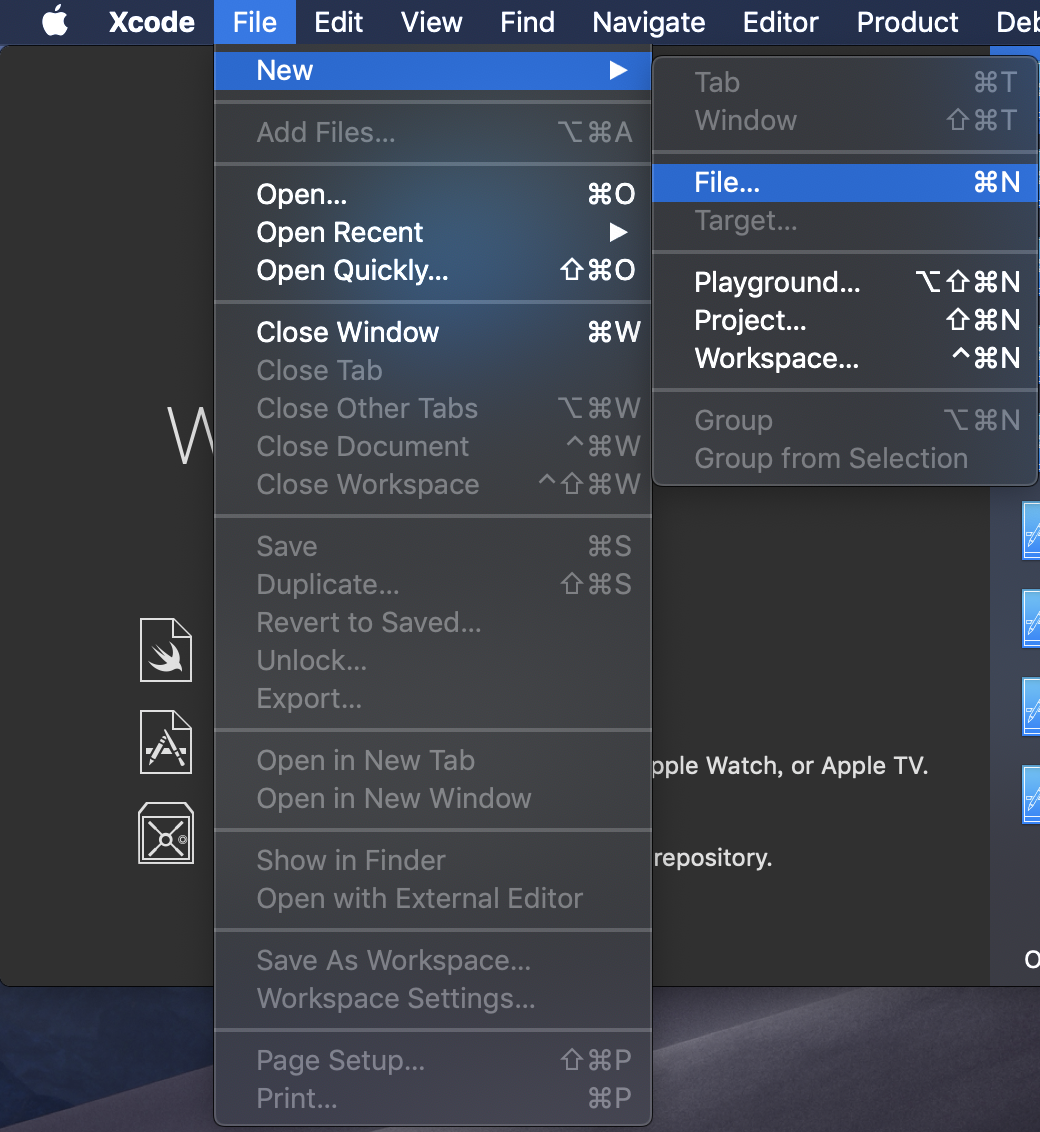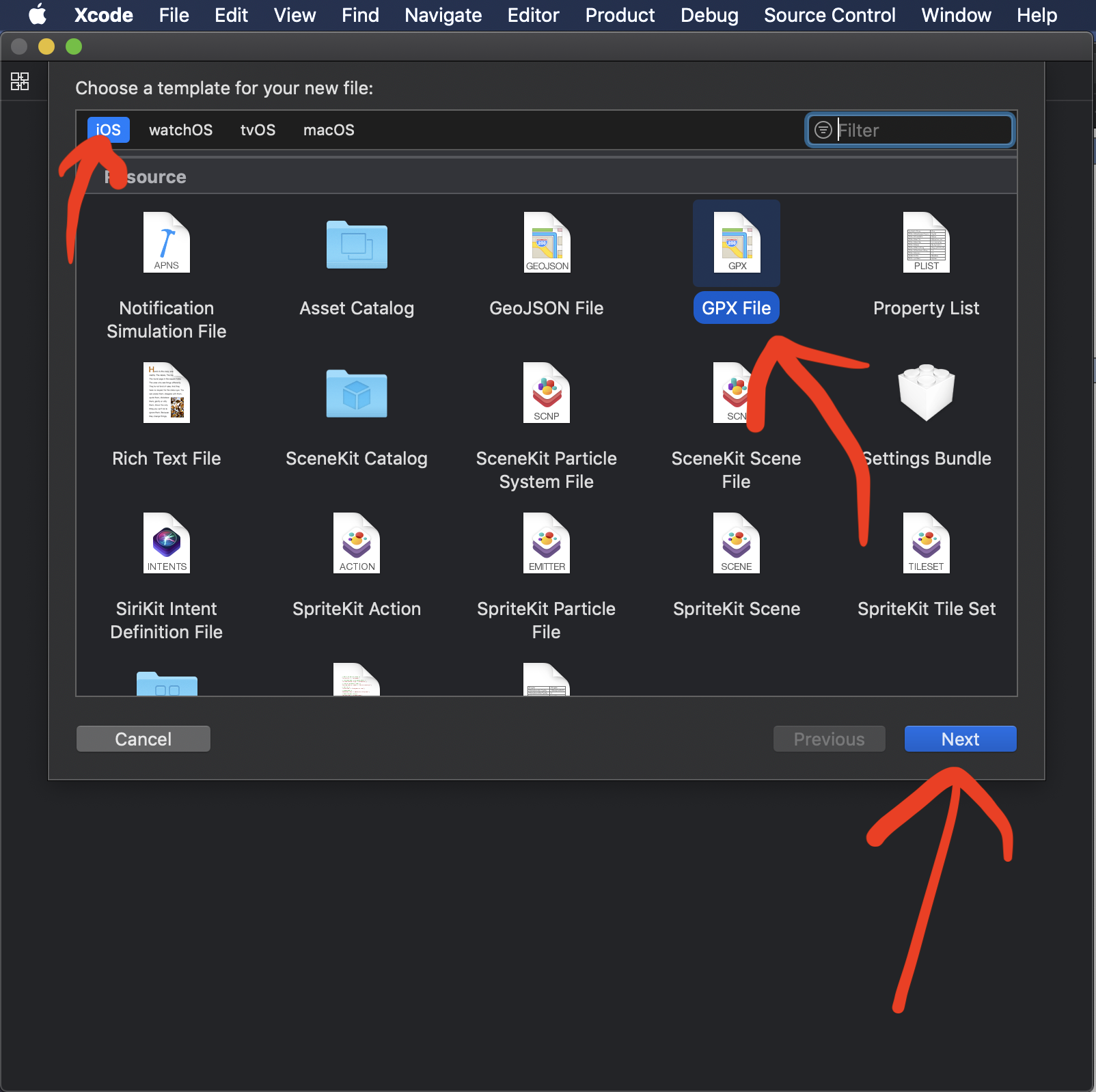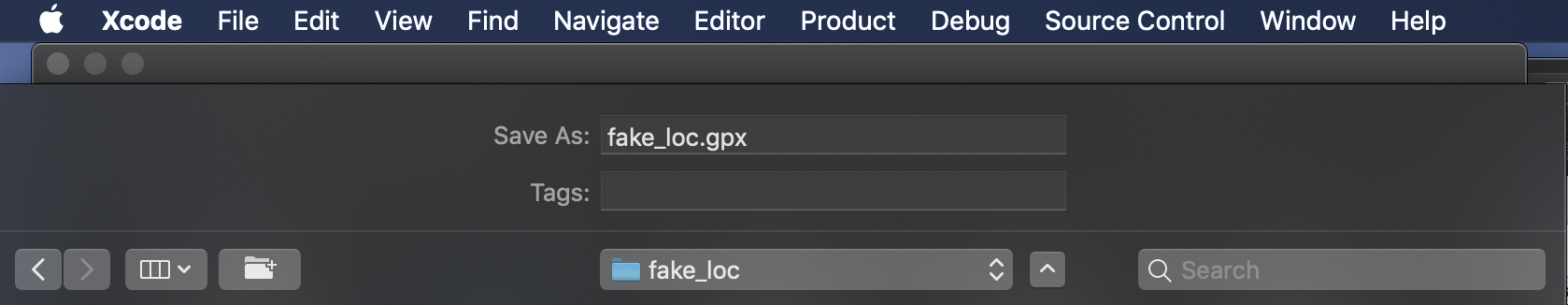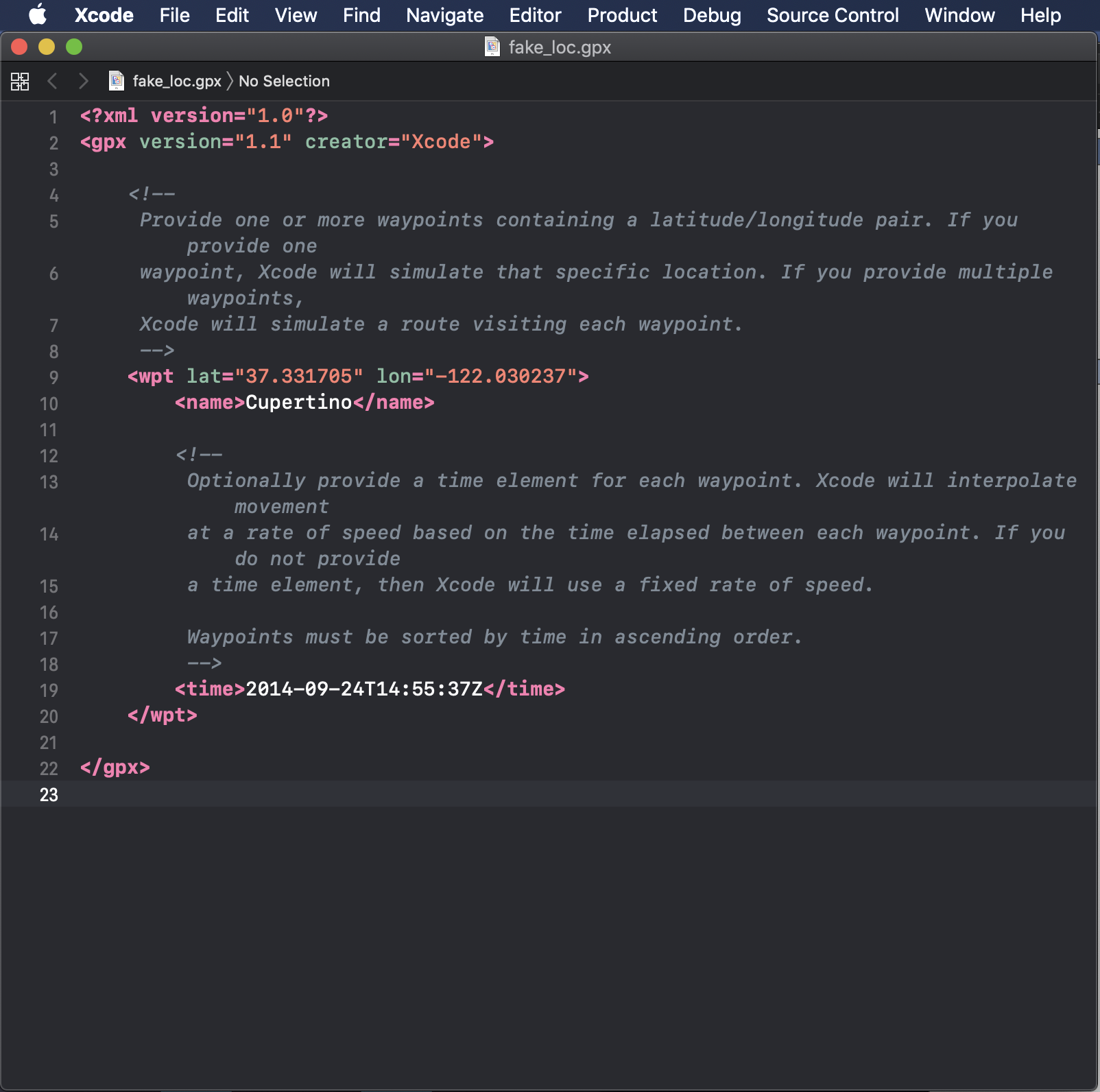“提供一个或多个包含纬度/经度对的点

如果提供一个点，Xcode将模拟那个特定的点

如果提供多个点，Xcode将依次模拟每一个点”

“可以选择为每一个坐标点添加一个时间标签可，Xcode可以根据时间标签的顺序依次移动到每一个坐标点，并根据时间点来插入速度X

如果不提供一个时间标签，那么Xcode将使用一个固定的速度

航路点必须按时间升序排序

## 3.使用Python来自动生成一系列轨迹点

mac下python与sublime text3的环境配置在第一篇随笔中有记录

<?xml version="1.0"?>
<gpx version="1.1" creator="Xcode">
<wpt lat="坐标点经度1" lon="坐标点纬度1">
</wpt>

<wpt lat="坐标点经度2" lon="坐标点纬度2">
</wpt>

<wpt lat="坐标点经度3" lon="坐标点纬度3">
</wpt>

#......
#以此类推
</gpx>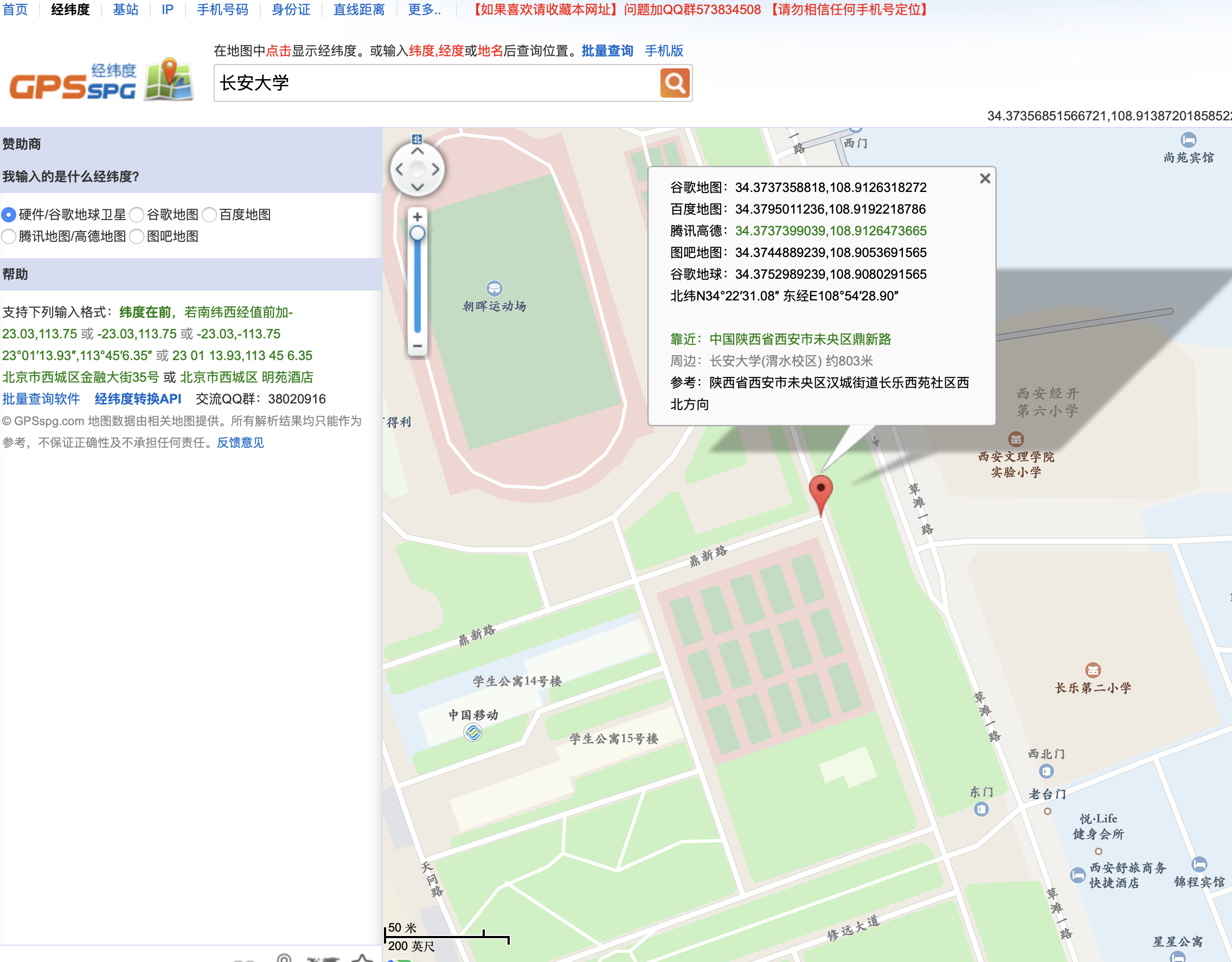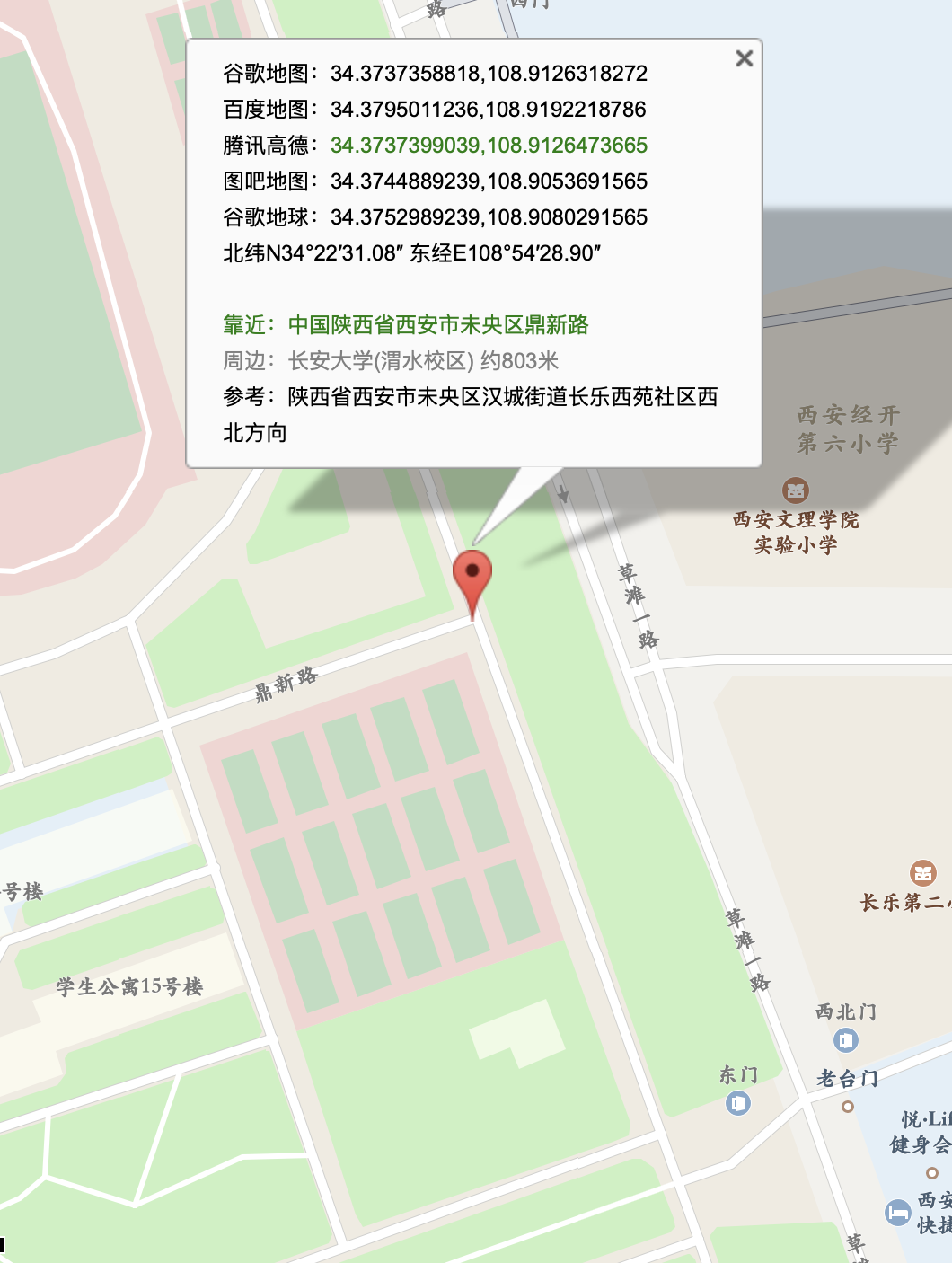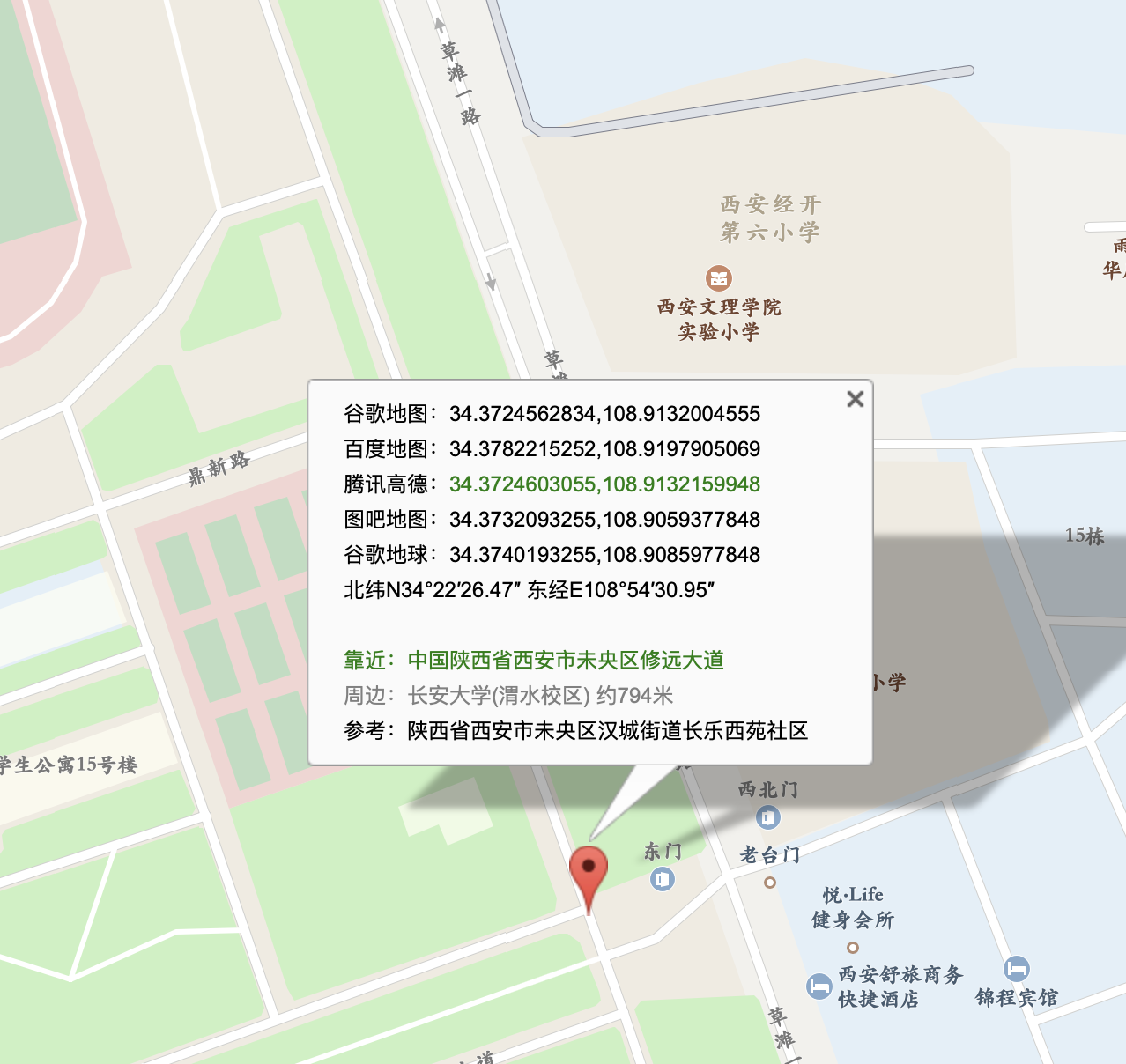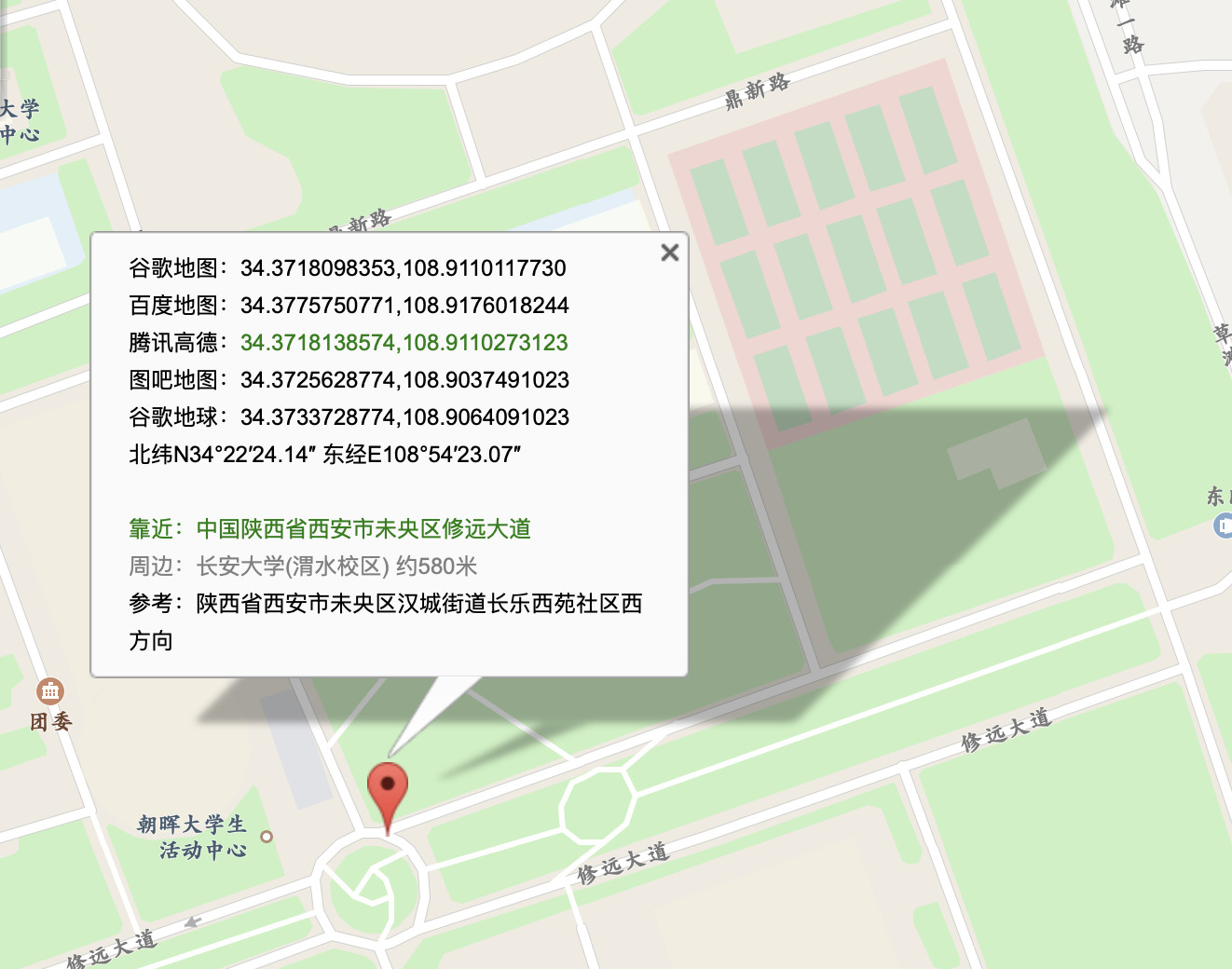lat：34.3752989239

lon：108.9080291565

lat：34.3740193255

lon：108.9085977848

lat：34.3733728774

lon：108.9064091023例如从北到南生成100个点

import random

#初始点信息
a1 = 34.3752989239;
b1 = 108.9080291565;
#中间点信息
a2 = 34.3740193255;
b2 = 108.9085977848;

for i in range( 1,1000 ):
while( a1 >= a2 ):        # 循环条件 经度未到达中间点时执行
xa = round( random.uniform(0.2, 7) )    # 随机数，用于模拟人跑动
xb = round( random.uniform(12, 15) )
# 竖直移动
a1 = round( a1 - 0.0000072 * xa , 10 )
b1 = round( b1 + 0.0000008 * xb , 10 )
# 输出信息
print( '<wpt lat="' + str( a1 ) + '"' + ' ' + 'lon="' + str( b1 ) + '">' )
print( '</wpt>' )

for i in range( 1,1000 ):
xa = round( random.uniform(2, 8) )
xb = round( random.uniform(1, 3) )
# 水平移动
a2 = round( a2 - 0.000003 * xa, 10 )
b2 = round( b2 - 0.000025 * xb, 10 )
print( '<wpt lat="' + str( a2 ) + '"' + ' ' + 'lon="' + str( b2 ) + '">' )
print( '</wpt>' )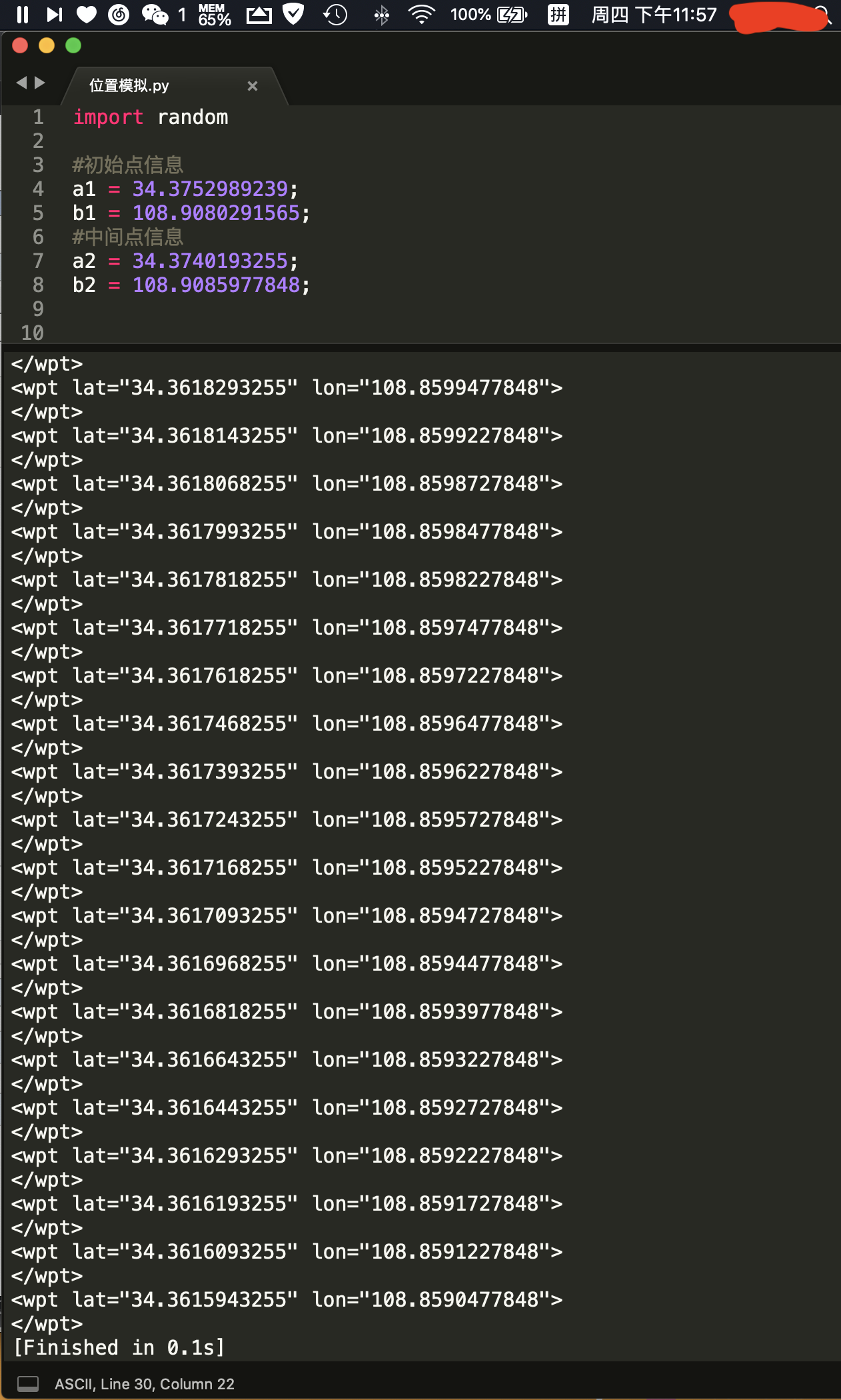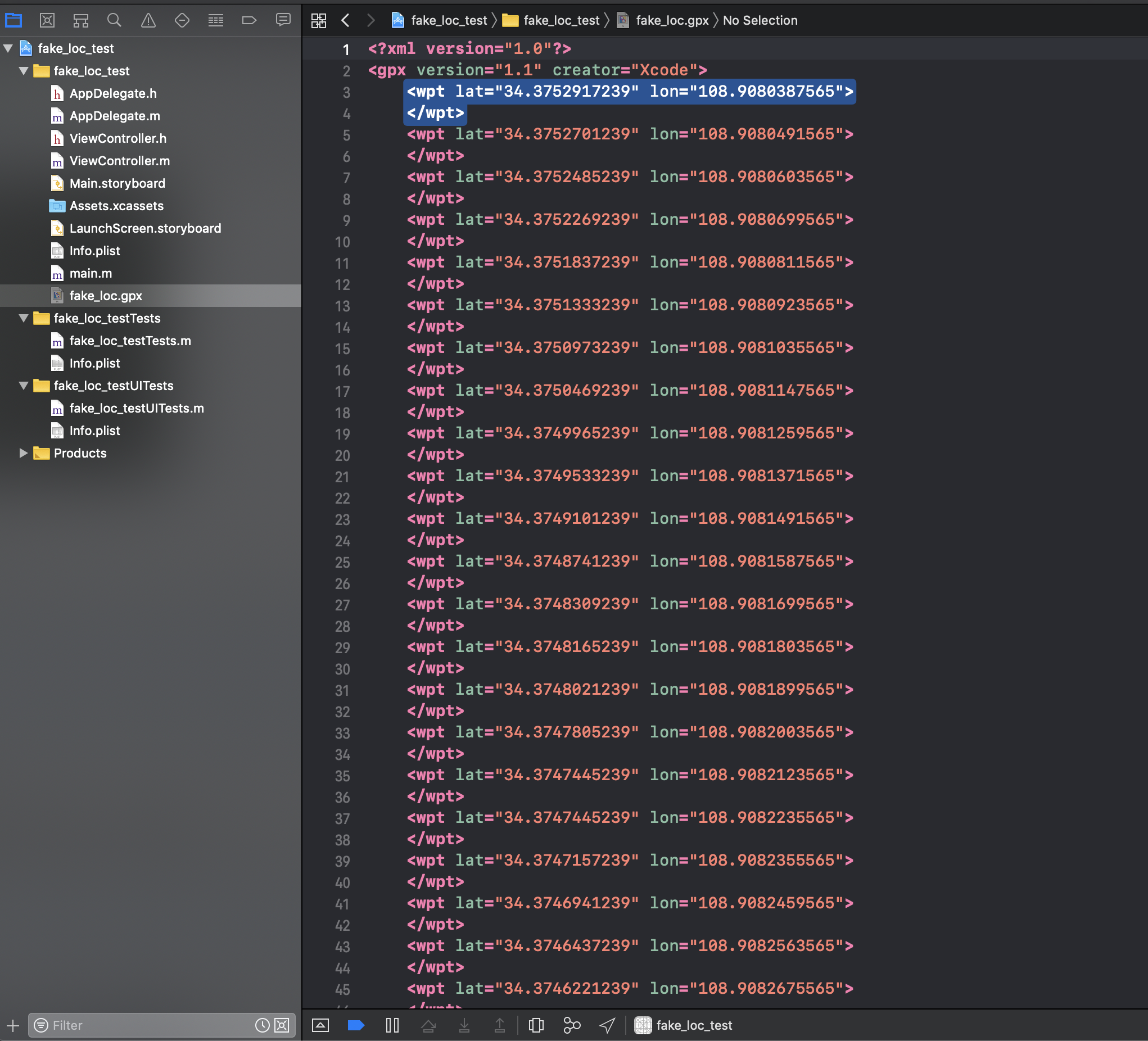## 4.开始位置模拟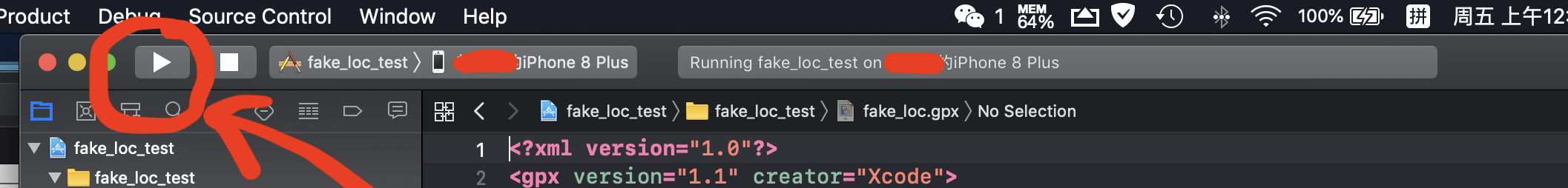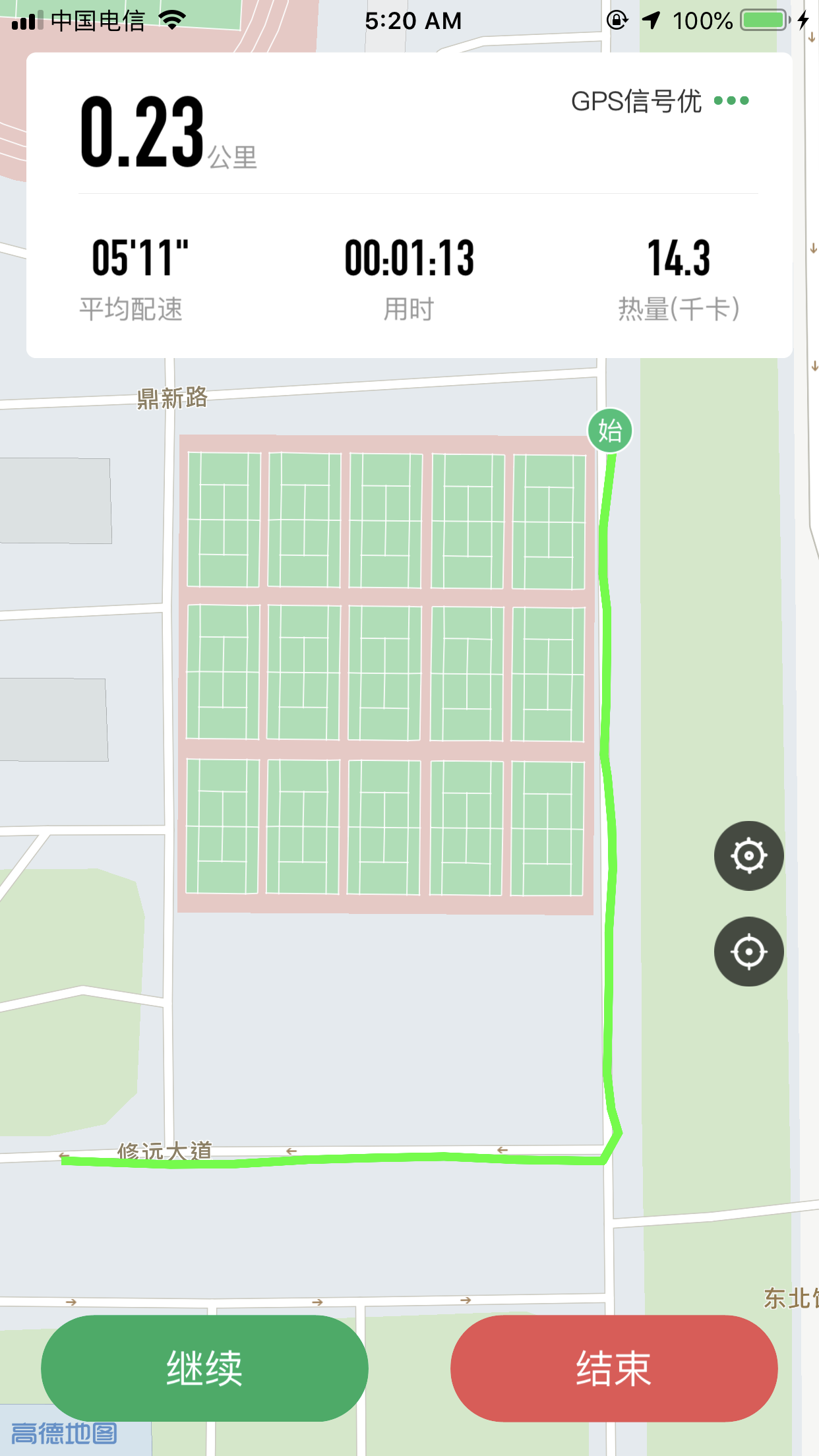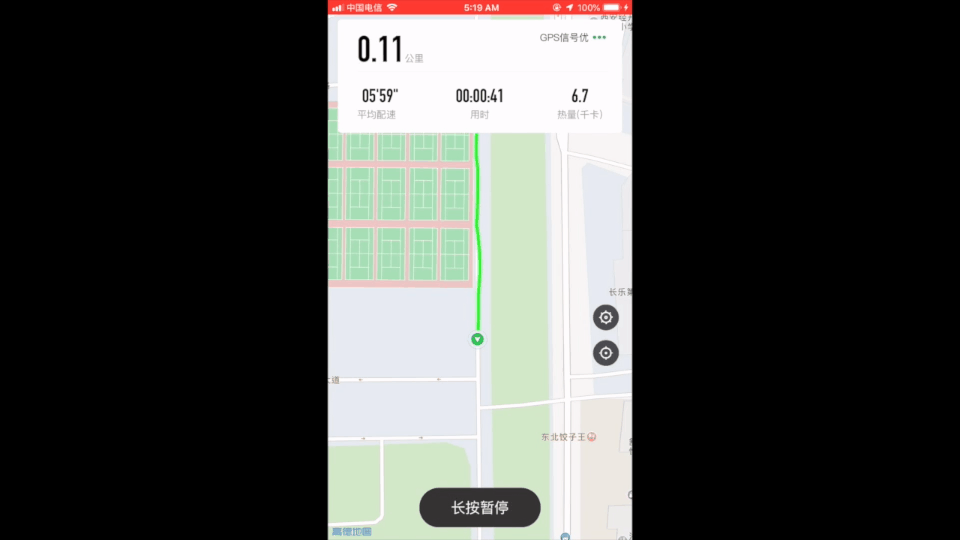## 5.总结

posted @ 2019-07-12 00:25 Joeric 阅读(...) 评论(...) 编辑 收藏